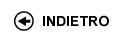Politecnico di Torino
04KWQLI, 04KWQJM, 04KWQLJ, 04KWQLL, 04KWQLM, 04KWQLS, 04KWQLX, 04KWQLZ, 04KWQMA, 04KWQMB, 04KWQMC, 04KWQMH, 04KWQMK, 04KWQMO, 04KWQMQ, 04KWQOD, 04KWQPC, 04KWQPI, 04KWQPL
Mathematical analysis I
Corso di Laurea in Ingegneria Dell'Autoveicolo (Automotive Engineering) - Torino
Corso di Laurea in Ingegneria Meccanica (Mechanical Engineering) - Torino
Corso di Laurea in Ingegneria Delle Telecomunicazioni (Telecommunications Engineering) - Torino
Espandi...
 Docente Qualifica Settore Lez Es Lab Tut Anni incarico Boieri Paolo 60 40 0 0 7 Boieri Paolo 60 40 0 0 7 Vaccarino FrancescoA2 MAT/03 60 40 0 0 2
 SSD CFU Attivita' formative Ambiti disciplinari MAT/05 10 A - Di base Matematica, informatica e statistica
Esclusioni:
16ACF
 Presentazione This course represents a bridge between high school and college ways of teaching and learning. The main goal of the course is to provide the students with tools to understand and elaborate logical arguments, from simple to more elaborate ones, and to introduce the elements of differential and integral calculus for functions of one variable with applications to ordinary differential equations of the first order and of the second order linear case. Complex numbers will also be introduced and applied to the solutions of linear equations of the second order with constant coefficients. Risultati di apprendimento attesi Ability to follow a chain of logical arguments. Essentials of differential and integral calculus for functions of one variable. Computational ability. Prerequisiti / Conoscenze pregresse Numbers, equations and inequalities, analytical geometry and trigonometry. First properties of elementary functions. Programma Preliminaries: sets and their operations. Number sets, maxima, minima and extrema. Completeness properties of real numbers and consequences. Functions: surjectivity and injectivity; composition of functions, inverse functions. Functions of a real variable: elementary functions, monotonic functions and inverse functions. (About 15 hours) Limits and continuity: theory of limits of functions and sequences. Continuous functions and related theorems. Order and comparison theorems. Algebra of limits. Indeterminate forms. Local comparison of functions. Landau symbols. Infinite and infinitesimal functions. Order of an infinity and of an infinitesimal, principal part (with respect to a test function). Asymptotes. The number e. Trigonometric and exponential fundamental limits. Functions which are continuous on an interval: existence of zeros and of maxima and minima. (About 24 hours) Derivatives: geometrical and physical meaning. Computation of derivatives. List of fundamental derivatives. Derivatives and continuity. Lack of derivatives, extremal and critical points. Fermat Theorem. Functions with derivatives on an interval and fundamental theorems of differential calculus (Rolle and Lagrange theorems) and consequences. de L'Hospital rule. Taylor formula and fundamental MacLaurin formulas. Use of Taylor expansions in the local analysis of functions: comparison, extrema and convexity. (About 23 hours) Primitives: rules of computation; primitives of rational functions. Indefinite integral. Riemann integral and its properties: monotonicity, additivity, linearity. Integral mean value. Classes of integrable functions. Fundamental theorem of integral calculus: relations between definite integrals and primitives. Improper integrals: definitions and convergence tests. (About 21 hours) Complex numbers and differential equations: algebraic and trigonometric forms of complex numbers. Real and imaginary parts, modulus and argument. Roots of complex numbers; Fundamental theorem of Algebra. Exponentials of complex numbers and Euler formulas. Ordinary differential equations: Cauchy problem. First order ordinary differential equations, with separable variables or linear. Second order differential equations, linear with constant coefficients. (About 17 hours) Organizzazione dell'insegnamento Both lessons and exercise classes will be given. Testi richiesti o raccomandati: letture, dispense, altro materiale didattico The teacher at lessons will suggest textbooks, from the following list. A. Bacciotti, Limiti, derivate, integrali (funzioni reali di una variabile reale), Celid, Torino 2006 C. Canuto, A. Tabacco, Mathematical Analysis 1, Springer-Verlag Italia, Milano 2008 L. Pandolfi, Analisi Matematica 1, Bollati-Boringhieri, Torino 1998 Usefull material can be downloaded from the WEB page of the Politecnico di Torino, and from the WEB pages of the courses. Criteri, regole e procedure per l'esame Written exams and possibly an oral examination. Orario delle lezioni Statistiche superamento esami Programma definitivo per l'A.A.2012/13© Politecnico di Torino
Corso Duca degli Abruzzi, 24 - 10129 Torino, ITALY